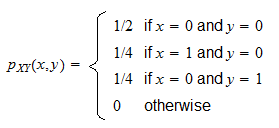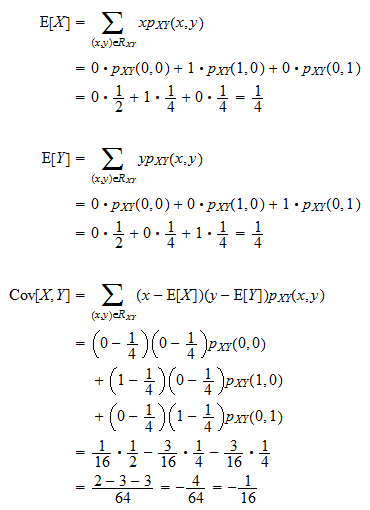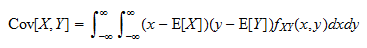StatLect

# Covariance formula

The covariance between two random variablesandcan be computed using the definition of covariance:where the capital letterindicates the expected value operator.## Formula for discrete variables

When the two random variables are discrete, the above formula can be written aswhereis the set of all couples of values ofandthat can possibly be observed andis the probability of observing a specific couple. This sum is a weighted average of the products of the deviations of the two random variables from their respective means.

To see how to apply this formula, read some Solved exercises.

## Formula for continuous variables

When the two random variables, taken together, form a continuous random vector, the formula can be expressed as a double integral:whereis the joint probability density function ofand.

To see how to apply this formula, read some Solved exercises.

## A simple covariance formula

Using the formulae above to compute covariance can sometimes be tricky. This is the reason why the following simpler (and equivalent) covariance formula is often used:For instance, this formula is straightforward to use when we know the joint moment generating function ofand. Taking partial derivatives of the joint moment generating function, we can derive the moments,andand then plug their values in this formula.

## More details

In the lecture entitled Covariance you can find more details about this formula, including a proof of it and some exercises.